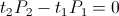Thread: Eric P. Dollard View Single PostRauiSenior Member Join Date: Dec 2008 Posts: 286
Kokomoj0,
I do not get what you mean by needle in a haystack definition as it seems we both understand what I mean when I say magnify or you wouldn't say that the voltage in a 1:2 transformer would be magnified and be double what appears on the primary. What's the problem? You are the one who seems to be on a word-smithing game. The word power was around in 1901 so they must have thought of electrical power as a different quantity to mechanical and thus labeled it as different in the same way that work and energy are different. The difference is only slight but it is real.

I am not actually saying this is a university course just giving an analogy as to why I choose to use the words that it was taught to me in. In the same way that if you were trying to explain something to someone you would use the same words you were taught in or the same words that the resources you are giving the student to study.

If you want to get your understanding of Tesla from the source then you need to understand what the source is saying. Secondary sources will often have a different take on the material to what is intended by the original author which is why I don't start changing words.

Like I've said that is the problem when modern science dawdles behind turn-of-the-century because when people want to go back they will always have to deal with changing ideas/words. I understand your beef with the words but it's really not THAT hard if you just read Eric's transmissions because I feel he defines the terms pretty well. In fact had you read Eric's transmissions you'd see that he clearly defined activity;
Quote:
 Thus the dimensional resultant of the union of the pair of dimensional laws is PLANCKS per SECOND SQUARED. We will call this the electrical ACTIVITY, also known as the electrical power, P. Hence the dimensional relation
I personally don't use the word activity often and when I do it's usually tied in with the word power as to help teach people to interpret the writings themselves. Obviously you don't see this as important but I do.

Geometric_Algebra,
That is an interesting point you raise. I see two distinct time periods here though where you see one. Here I am taking the ratio of power measured across a resistor to charge a capacitor to power measured across a different resistor to which a capacitor was discharged. The whole point of my experiment was that the power consumed in charging the capacitor will remain the same and so if the power measured across the resistor changes for different values of R we have a difference in power which could be expressed as a ratio.

With the situation you are talking about are you talking about the ratio between the power in the dielectric field being discharged to the magnetic field being charged and visa versa?
Looking to Eric's posts Four Quadrant Energy Exchange in Magnetic & Dielectric Fields of Induction now look at figure 3 and 4.
Quote:
 Thru adjustment of the time rate of charge, and the time rate of discharge, involved in Energy Transfer into, or out of, a Field of Induction, any magnitude of Electrical Activity, P, can be developed from a given quantity of stored Energy, W, Fig 3, Fig 4.
From this quote you maybe able to see where I got my understanding of it from.

So when we charge the capacitor we have an energy transfer into a field of induction, this is one time period, t1, and when we discharge the capacitor we have an energy transfer out of this field of induction, the second time period, t2. The two time periods of this exchange, that is t1/t2, give us are power magnification ratio.
So now for some mathematics. Since energy is conserved if we have a changing power and time periods the following condition must be true;Joules
So taking the ratio of the powers or time periods we get;This is how I define power magnification, if anyone else has another definition go right ahead and explain it. It is important we get this issue sorted.

EDIT: Just seen your next post G_A and it seems our definitions of power magnification are the same but we disagree as to whether or not it can be applied to the situation I've presented, do you agree?

This is Tesla's power magnification setup notice that it just charges a capacitor and then the capacitor is discharged to the load through the spark gap.More on this here; Nikola Tesla On His Work With Alternating Currents -- Chapter IV

EDIT EDIT: I think I can see where the confusing stems from. I was assuming everyone knew that in my experiment the two time frames were dependant on the resistors and so I calculated the power magnification factor from the power and compared it to the resistors rather than the time because the time rates change with the resistance in my experiment.

Raui
__________________
Scribd account; http://www.scribd.com/raui

Last edited by Raui; 04-19-2012 at 04:43 AM.